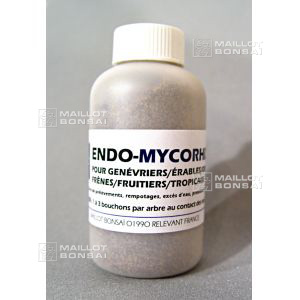##### The Japanese Bonsai specialist
Direct order Contact Help / Services Newsletter# Endo mycorhize tropical and fruit bonsai treatment

› Bonsai soil and fertiliser › Bonsai maintenanceref. : 5262

10,00

Available quantity : 61Order

###### Description

Small bottle 100Gr. This ENDO mycorhize treatment contains a different mix to our other product and is meant for fertilising orchids, maple, juniper, ash, elm, fruit and tropical bonsai trees. See the list below.

It's especially good for bonsai with root problems such as excess water or root rot. It's highly recommended that you use this product when potting trees found in the forest (yamadori) that you want to train into a bonsai.

Benefits: mycorhize may reduce infection by bacteria and fungi that cause plant diseases. It can also help fix vital nutrients, promoting plant growth.

How to use: Use 1 to 3 capfuls per tree, make four or five holes in the earth, near the trunk and surface roots (not in the bottom of the pot), and add some powder to each. Re-cover the holes with earth afterwards.

Species:
Acer (Maple)
Monkey Puzzle
Catalpa

Juniper – Cypress – Taxus - Thuja

Ericaceae

Azalea – heather - rhododendron
Ginkgo biloba
Magnolia – tulip tree
Oleaceae

Forsythia

Ash - jasmine - Ligustrum - lilac - olive
Orchids
Plane tree
Podocarpus
Cherry tree, plum tree – quince tree - Cotoneaster – cherry laurel (Prunus laurocerasus) – pear tree (Pyrus) – apple tree (Malus) – rose bush (Rosa) – rowan tree
Sequoia or redwood
Elms
Nettle or hackberry tree (Micocoulier) - elm (Orme) - Zelkova (Siberian elm)

Taxodiaceae

#tree 10.4 #bonsai 7.6 #mycorhize 4.9 #treatment 3.9 #tropical 3.8 #fruit 3.5 #that 3.4 #endo 3.4 #maintenance 3.1 #product 2.7

Formule
(( ROUND((CHAR_LENGTH(b.article_nom)-CHAR_LENGTH(REPLACE(b.article_nom, 'tree', '')))/LENGTH('tree')) + ROUND((CHAR_LENGTH(b.article_description)-CHAR_LENGTH(REPLACE(b.article_description, 'tree', '')))/LENGTH('tree')) ) * 10.4) + (( ROUND((CHAR_LENGTH(b.article_nom)-CHAR_LENGTH(REPLACE(b.article_nom, 'bonsai', '')))/LENGTH('bonsai')) + ROUND((CHAR_LENGTH(b.article_description)-CHAR_LENGTH(REPLACE(b.article_description, 'bonsai', '')))/LENGTH('bonsai')) ) * 5.6) + (( ROUND((CHAR_LENGTH(b.article_nom)-CHAR_LENGTH(REPLACE(b.article_nom, 'mycorhize', '')))/LENGTH('mycorhize')) + ROUND((CHAR_LENGTH(b.article_description)-CHAR_LENGTH(REPLACE(b.article_description, 'mycorhize', '')))/LENGTH('mycorhize')) ) * 4.9) + (( ROUND((CHAR_LENGTH(b.article_nom)-CHAR_LENGTH(REPLACE(b.article_nom, 'treatment', '')))/LENGTH('treatment')) + ROUND((CHAR_LENGTH(b.article_description)-CHAR_LENGTH(REPLACE(b.article_description, 'treatment', '')))/LENGTH('treatment')) ) * 3.9) + (( ROUND((CHAR_LENGTH(b.article_nom)-CHAR_LENGTH(REPLACE(b.article_nom, 'tropical', '')))/LENGTH('tropical')) + ROUND((CHAR_LENGTH(b.article_description)-CHAR_LENGTH(REPLACE(b.article_description, 'tropical', '')))/LENGTH('tropical')) ) * 3.8) + (( ROUND((CHAR_LENGTH(b.article_nom)-CHAR_LENGTH(REPLACE(b.article_nom, 'fruit', '')))/LENGTH('fruit')) + ROUND((CHAR_LENGTH(b.article_description)-CHAR_LENGTH(REPLACE(b.article_description, 'fruit', '')))/LENGTH('fruit')) ) * 3.5) + (( ROUND((CHAR_LENGTH(b.article_nom)-CHAR_LENGTH(REPLACE(b.article_nom, 'that', '')))/LENGTH('that')) + ROUND((CHAR_LENGTH(b.article_description)-CHAR_LENGTH(REPLACE(b.article_description, 'that', '')))/LENGTH('that')) ) * 3.4) + (( ROUND((CHAR_LENGTH(b.article_nom)-CHAR_LENGTH(REPLACE(b.article_nom, 'endo', '')))/LENGTH('endo')) + ROUND((CHAR_LENGTH(b.article_description)-CHAR_LENGTH(REPLACE(b.article_description, 'endo', '')))/LENGTH('endo')) ) * 3.4) + (( ROUND((CHAR_LENGTH(b.article_nom)-CHAR_LENGTH(REPLACE(b.article_nom, 'orchids', '')))/LENGTH('orchids')) + ROUND((CHAR_LENGTH(b.article_description)-CHAR_LENGTH(REPLACE(b.article_description, 'orchids', '')))/LENGTH('orchids')) ) * 2.7) + (( ROUND((CHAR_LENGTH(b.article_nom)-CHAR_LENGTH(REPLACE(b.article_nom, 'juniper', '')))/LENGTH('juniper')) + ROUND((CHAR_LENGTH(b.article_description)-CHAR_LENGTH(REPLACE(b.article_description, 'juniper', '')))/LENGTH('juniper')) ) * 2.7)

## Secure payment## Delivery

Our logistic partners :04 74 55 23 48
Pépinière MAILLOT-BONSAÏ
Le Bois Frazy
01990 RELEVANT - FRANCE
on appointment